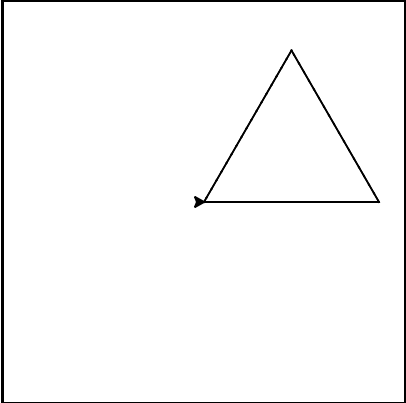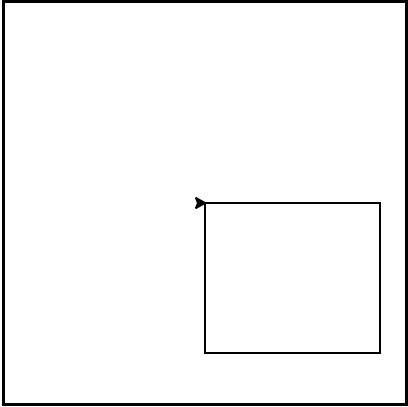# How to Think Like a Computer Scientist: The PreTeXt Interactive Edition

## Section4.6Iteration Simplifies our Turtle Program

To draw a square we’d like to do the same thing four times — move the turtle forward some distance and turn 90 degrees. We previously used 8 lines of Python code to have alex draw the four sides of a square. This next program does exactly the same thing but, with the help of the for statement, uses just three lines (not including the setup code). Remember that the for statement will repeat the forward and left four times, one time for each value in the list.
While “saving some lines of code” might be convenient, it is not the big deal here. What is much more important is that we’ve found a “repeating pattern” of statements, and we reorganized our program to repeat the pattern. Finding the chunks and somehow getting our programs arranged around those chunks is a vital skill when learning How to think like a computer scientist.
The values [0,1,2,3] were provided to make the loop body execute 4 times. We could have used any four values. For example, consider the following program.
In the previous example, there were four integers in the list. This time there are four strings. Since there are four items in the list, the iteration will still occur four times. aColor will take on each color in the list. We can even take this one step further and use the value of aColor as part of the computation.
In this case, the value of aColor is used to change the color attribute of alex. Each iteration causes aColor to change to the next value in the list.
The for-loop is our first example of a compound statement. Syntactically a compound statement is a statement. The level of indentation of a (whole) compound statement is the indentation of its heading. In the example above there are five statements with the same indentation, executed sequentially: the import, 2 assignments, the whole for-loop, and wn.exitonclick(). The for-loop compound statement is executed completely before going on to the next sequential statement, wn.exitonclick().
Mixed up program

### Checkpoint4.6.1.

The following program uses a turtle to draw a triangle as shown to the left:But the lines are mixed up. The program should do all necessary set-up and create the turtle. After that, iterate (loop) 3 times, and each time through the loop the turtle should go forward 175 pixels, and then turn left 120 degrees. After the loop, set the window to close when the user clicks in it.
Drag the blocks of statements from the left column to the right column and put them in the right order with the correct indention. Click on <i>Check Me</i> to see if you are right. You will be told if any of the lines are in the wrong order or are incorrectly indented.
Mixed up program

### Checkpoint4.6.2.

The following program uses a turtle to draw a rectangle as shown to the left:But the lines are mixed up. The program should do all necessary set-up and create the turtle. After that, iterate (loop) 2 times, and each time through the loop the turtle should go forward 175 pixels, turn right 90 degrees, go forward 150 pixels, and turn right 90 degrees. After the loop, set the window to close when the user clicks in it.
Drag the blocks of statements from the left column to the right column and put them in the right order with the correct indention. Click on <i>Check Me</i> to see if you are right. You will be told if any of the lines are in the wrong order or are incorrectly indented.

### Checkpoint4.6.3.

In the following code, how many lines does this code print?
for number in [5, 4, 3, 2, 1, 0]:
print("I have", number, "cookies.  I'm going to eat one.")

• 1
• The loop body prints one line, but the body will execute exactly one time for each element in the list [5, 4, 3, 2, 1, 0].
• 5
• Although the biggest number in the list is 5, there are actually 6 elements in the list.
• 6
• The loop body will execute (and print one line) for each of the 6 elements in the list [5, 4, 3, 2, 1, 0].
• 10
• The loop body will not execute more times than the number of elements in the list.

### Checkpoint4.6.4.

How does python know what statements are contained in the loop body?
• They are indented to the same degree from the loop header.
• The loop body can have any number of lines, all indented from the loop header.
• There is always exactly one line in the loop body.
• The loop body may have more than one line.
• The loop body ends with a semi-colon (;) which is not shown in the code above.
• Python does not need semi-colons in its syntax, but relies mainly on indentation.

### Checkpoint4.6.5.

In the following code, what is the value of number the second time Python executes the loop?
for number in [5, 4, 3, 2, 1, 0]:
print("I have", number, "cookies.  I'm going to eat one.")

• 2
• Python gives number the value of items in the list, one at a time, in order (from left to right). number gets a new value each time the loop repeats.
• 4
• Yes, Python will process the items from left to right so the first time the value of number is 5 and the second time it is 4.
• 5
• Python gives number the value of items in the list, one at a time, in order. number gets a new value each time the loop repeats.
• 1
• Python gives number the value of items in the list, one at a time, in order (from left to right). number gets a new value each time the loop repeats.

### Checkpoint4.6.6.

Consider the following code:
for aColor in ["yellow", "red", "green", "blue"]:
alex.forward(50)
alex.left(90)

What does each iteration through the loop do?
• Draw a square using the same color for each side.
• The question is not asking you to describe the outcome of the entire loop, the question is asking you about the outcome of a **single iteration** of the loop.
• Draw a square using a different color for each side.
• Notice that aColor is never actually used inside the loop.
• Draw one side of a square.
• The body of the loop only draws one side of the square. It will be repeated once for each item in the list. However, the color of the turtle never changes.